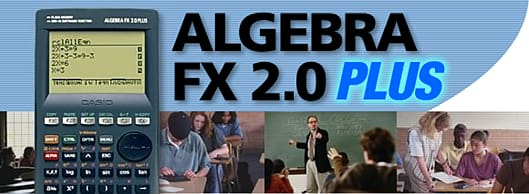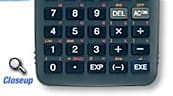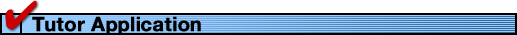Students can study how to solve algebra problems as ALGEBRA FX 2.0 PLUS shows each step to arrive at the final solution, in a similar way as a teacher does it in class. It works under three different modes.Auto Mode In this mode, the solution is produced automatically step by step, so students can learn how to solve the problem.Manual Mode In this mode, students can manipulate equations using algebra commands and try to solve the problems by themselves.Verify Mode In this mode, students can verify whether the solution they have arrived at is correct or not.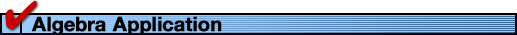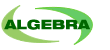This application helps students manipulate equations. They can, for example, expand or simplify equations by themselves in an attempt to arrive at the correct solutions.After they learn how to solve the problem in Tutor application, they can make use of this application to master how to solve the algebra problems.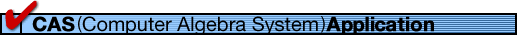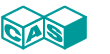Using the Computer Algebra System (CAS), students can factor expressions, find limits of functions and calculate derivatives, integration and Taylor series expressions.Casio's CAS is based on the Casio's original algebra system and enhanced by our R & D team, Professor John Kenelly and other math instructors, adopting teachers' advice and suggestion.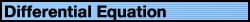When this software is installed in a graphic calculator, it can calculate an approximate solution of a differential equation with given initial values numerically or solve it graphically.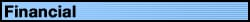Installing this software makes the operations of Depreciation, Bond Calculation, and TVM Graph available, in addition to the Financial operations of the CFX-9850GB PLUS.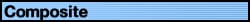Installing the add-in will give you a means of analyzing composite functions.Operations of tests, confidence intervals and probability distributions that can be used in statistics for the elementary education in high schools, colleges and universities are available as well as the built-in statistic features of the ALGEBRA FX Series.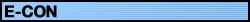Enables you to carry out the sophisticated settings automatically according to the guidance displayed on the screen, so that you can analyze the phenomena of the nature using the data analyzer EA-100 without writing a program, conduct experiments, and analyze the results easily.By connecting the ALGEBRA FX 2.0 PLUS to a PC with the dedicated cable (optional), you can transfer a variety of add-in applications to the ALGEBRA FX 2.0 PLUS using a special installer software.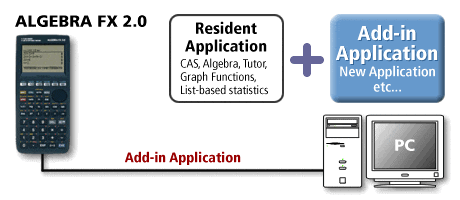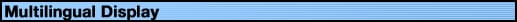The ALGEBRA FX 2.0 PLUS can display messages in English, Spanish, German, French and Italian, and it is very easy to switch from one language to other language.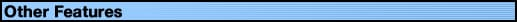[ 146,000 Bytes ] [ 768KB Flash Memory ] [ 21characters by 8 lines ] [ 10 DIGITS or 10+2 DIGITS ] [ ICON MENU ] [ DOT MATRIX ]Large display (128 * 64 dots)Graphic functions & Graph solve functionsDynamic graphDual graph(Graph & Table,Graph & Graph)Conics function graphComplex functionsList function & List-based statisticsStatistic calculations & graphsBASIC-like program functionsLinear equations from 2 to 30 unknowns2 to 30 order equationsMatrix operations with complex numbersIncludes a connecting cable for data transfer between two unitsData communicationsPower source: Main-Four AAA size batteries(R03/LR03)Backup-One lithium battery(CR2032)Battery life: Main-Approx.140 hours with R03/230 hours with LR03(continuous display of main menu)Dimensions:19.5H * 82W * 178D mmWeight:Approx.213gHow to make use of the program resources made using the 9850 Series with the ALGEBRA FX 2.0 PLUSIf you have switched from the 9850 Series to the ALGEBRA FX 2.0 PLUS, you can use the programs made using the 9850 Series with the ALGEBRA FX 2.0 PLUS. The program can be transferred to the ALGEBRA FX 2.0 PLUS via the PC Link software. However, you cannot transfer the programs by connecting the 9850 Series and the ALGEBRA FX 2.0 PLUS directly using the attached SB-62.